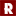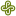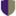Algebraic Geometry Commons™

223 Full-Text Articles 268 Authors 56,862 Downloads69 Institutions

All Articles in Algebraic Geometry

223 full-text articles. Page 4 of 9.

Tropical Convexity Over Max-Min Semiring, 2016West Chester University of Pennsylvania

Tropical Convexity Over Max-Min Semiring, Viorel Nitica, Sergei Sergeev

Viorel Nitica

No abstract provided.

A Metric On Max-Min Algebra, 2016University of Oregon

A Metric On Max-Min Algebra, Jonathan Eskeldson, Miriam Jaffe, Viorel Nitica

Viorel Nitica

No abstract provided.

On Hyperplanes And Semispaces In Max-Min Convex Geometry, 2016West Chester University of Pennsylvania

On Hyperplanes And Semispaces In Max-Min Convex Geometry, Viorel Nitica, Sergeĭ Sergeev

Viorel Nitica

No abstract provided.

A Metric On Max-Min Algebra, 2016University of Oregon

A Metric On Max-Min Algebra, Jonathan Eskeldson, Miriam Jaffe, Viorel Nitica

Viorel Nitica

No abstract provided.

On Hyperplanes And Semispaces In Max-Min Convex Geometry, 2016West Chester University of Pennsylvania

On Hyperplanes And Semispaces In Max-Min Convex Geometry, Viorel Nitica, Sergeĭ Sergeev

Viorel Nitica

No abstract provided.

2016Rose-Hulman Institute of Technology

Topological And Hq Equivalence Of Prime Cyclic P-Gonal Actions On Riemann Surfaces, Sean A. Broughton

Mathematical Sciences Technical Reports (MSTR)

Two Riemann surfaces S1 and S2 with conformal G-actions have topologically equivalent actions if there is a homeomorphism h : S1 -> S2 which intertwines the actions. A weaker equivalence may be defined by comparing the representations of G on the spaces of holomorphic q-differentials Hq(S1) and Hq(S2). In this note we study the differences between topological equivalence and Hq equivalence of prime cyclic actions, where S1/G and S2/G have genus zero.

The Implicit Function Theorem And Free Algebraic Sets, 2016Washington University in St Louis

The Implicit Function Theorem And Free Algebraic Sets, Jim Agler, John E. Mccarthy

Mathematics Faculty Publications

We prove an implicit function theorem for non-commutative functions. We use this to show that if p ( X;Y ) is a generic non-commuting polynomial in two variables, and X is a generic matrix, then all solutions Y of p ( X;Y ) = 0 will commute with X

Klein Bottle Queries, 2016Georgia State University

Klein Bottle Queries, Austin Lowe

Georgia State Undergraduate Research Conference

No abstract provided.

Aspects Of Non-Commutative Function Theory, 2016Washington University in St Louis

Aspects Of Non-Commutative Function Theory, Jim Agler, John E. Mccarthy

Mathematics Faculty Publications

We discuss non commutative functions, which naturally arise when dealing with functions of more than one matrix variable.

The Log-Exponential Smoothing Technique And Nesterov’S Accelerated Gradient Method For Generalized Sylvester Problems, 2016Thua Thien Hue College of Education

The Log-Exponential Smoothing Technique And Nesterov’S Accelerated Gradient Method For Generalized Sylvester Problems, N. T. An, Daniel J. Giles, Nguyen Mau Nam, R. Blake Rector

Mathematics and Statistics Faculty Publications and Presentations

The Sylvester or smallest enclosing circle problem involves finding the smallest circle enclosing a finite number of points in the plane. We consider generalized versions of the Sylvester problem in which the points are replaced by sets. Based on the log-exponential smoothing technique and Nesterov’s accelerated gradient method, we present an effective numerical algorithm for solving these problems.

Minimizing Differences Of Convex Functions With Applications To Facility Location And Clustering, 2016Portland State University

Minimizing Differences Of Convex Functions With Applications To Facility Location And Clustering, Mau Nam Nguyen, R. Blake Rector, Daniel J. Giles

Mathematics and Statistics Faculty Publications and Presentations

In this paper we develop algorithms to solve generalized Fermat-Torricelli problems with both positive and negative weights and multifacility location problems involving distances generated by Minkowski gauges. We also introduce a new model of clustering based on squared distances to convex sets. Using the Nesterov smoothing technique and an algorithm for minimizing differences of convex functions called the DCA introduced by Tao and An, we develop effective algorithms for solving these problems. We demonstrate the algorithms with a variety of numerical examples.

Adinkras And Arithmetical Graphs, 2016Harvey Mudd College

HMC Senior Theses

Adinkras and arithmetical graphs have divergent origins. In the spirit of Feynman diagrams, adinkras encode representations of supersymmetry algebras as graphs with additional structures. Arithmetical graphs, on the other hand, arise in algebraic geometry, and give an arithmetical structure to a graph. In this thesis, we will interpret adinkras as arithmetical graphs and see what can be learned.

Our work consists of three main strands. First, we investigate arithmetical structures on the underlying graph of an adinkra in the specific case where the underlying graph is a hypercube. We classify all such arithmetical structures and compute some of the corresponding ...

Convexity Of Neural Codes, 2016Harvey Mudd College

Convexity Of Neural Codes, Robert Amzi Jeffs

HMC Senior Theses

An important task in neuroscience is stimulus reconstruction: given activity in the brain, what stimulus could have caused it? We build on previous literature which uses neural codes to approach this problem mathematically. A neural code is a collection of binary vectors that record concurrent firing of neurons in the brain. We consider neural codes arising from place cells, which are neurons that track an animal's position in space. We examine algebraic objects associated to neural codes, and completely characterize a certain class of maps between these objects. Furthermore, we show that such maps have natural geometric implications related ...

Topology Of The Affine Springer Fiber In Type A, 2016University of Massachusetts - Amherst

Topology Of The Affine Springer Fiber In Type A, Tobias Wilson

Doctoral Dissertations

We develop algorithms for describing elements of the affine Springer fiber in type

A for certain 2 g(C[[t]]). For these , which are equivalued, integral, and regular,

it is known that the affine Springer fiber, X, has a paving by affines resulting from

the intersection of Schubert cells with X. Our description of the elements of Xallow

us to understand these affine spaces and write down explicit dimension formulae. We

also explore some closure relations between the affine spaces and begin to describe the

moment map for the both the regular and extended torus action.

Algebraicity Of Rational Hodge Isometries Of K3 Surfaces, 2016University of Massachusetts Amherst

Algebraicity Of Rational Hodge Isometries Of K3 Surfaces, Nikolay Buskin

Doctoral Dissertations

Consider any rational Hodge isometry

$\psi:H^2(S_1,\QQ)\rightarrow H^2(S_2,\QQ)$ between any two K\"ahler $K3$

surfaces $S_1$ and $S_2$. We prove that the cohomology class of $\psi$ in $H^{2,2}(S_1\times S_2)$

is a polynomial in Chern classes of coherent analytic sheaves

over $S_1 \times S_2$. Consequently, the cohomology class of $\psi$ is algebraic

whenever $S_1$ and $S_2$ are algebraic.

2016University of Massachusetts - Amherst

Skein Theory And Algebraic Geometry For The Two-Variable Kauffman Invariant Of Links, Thomas Shelly

Doctoral Dissertations

We conjecture a relationship between the Hilbert schemes of points on a singular plane curve and the Kauffman invariant of the link associated to the singularity. Specifcally, we conjecture that the generating function of certain weighted Euler characteristics of the Hilbert schemes is given by a normalized specialization of the difference between the Kauffman and HOMFLY polynomials of the link. We prove the conjecture for torus knots. We also develop some skein theory for computing the Kauffman polynomial of links associated to singular points on plane curves.

Spherical Tropicalization, 2016University of Massachusetts Amherst

Spherical Tropicalization, Anastasios Vogiannou

Doctoral Dissertations

In this thesis, I extend tropicalization of subvarieties of algebraic tori over a trivially valued algebraically closed field to subvarieties of spherical homogeneous spaces. I show the existence of tropical compactifications in a general setting. Given a tropical compactification of a closed subvariety of a spherical homogeneous space, I show that the support of the colored fan of the ambient spherical variety agrees with the tropicalization of the closed subvariety. I provide examples of tropicalization of subvarieties of GL(n), SL(n), and PGL(n).

Quasi-Platonic Psl2(Q)-Actions On Closed Riemann Surfaces, 2015Rose-Hulman Institute of Technology

Quasi-Platonic Psl2(Q)-Actions On Closed Riemann Surfaces, Sean A. Broughton

Mathematical Sciences Technical Reports (MSTR)

This paper is the first of two papers whose combined goal is to explore the dessins d'enfant and symmetries of quasi-platonic actions of PSL2(q). A quasi-platonic action of a group G on a closed Riemann S surface is a conformal action for which S/G is a sphere and S->S/G is branched over {0, 1,infinity}. The unit interval in S/G may be lifted to a dessin d'enfant D, an embedded bipartite graph in S. The dessin forms the edges and vertices of a tiling on S by dihedrally symmetric polygons, generalizing the ...

Quasi-Platonic Psl2(Q)-Actions On Closed Riemann Surfaces, 2015Rose-Hulman Institute of Technology

Quasi-Platonic Psl2(Q)-Actions On Closed Riemann Surfaces, Sean A. Broughton

S. Allen Broughton

This paper is the first of two papers whose combined goal is to explore the dessins d'enfant and symmetries of quasi-platonic actions of PSL2(q). A quasi-platonic action of a group G on a closed Riemann S surface is a conformal action for which S/G is a sphere and S->S/G is branched over {0, 1,infinity}. The unit interval in S/G may be lifted to a dessin d'enfant D, an embedded bipartite graph in S. The dessin forms the edges and vertices of a tiling on S by dihedrally symmetric polygons, generalizing the ...

2015Washington University in St. Louis

Integrability And Regularity Of Rational Functions, Greg Knese

Mathematics Faculty Publications

Motivated by recent work in the mathematics and engineering literature, we study integrability and non-tangential regularity on the two-torus for rational functions that are holomorphic on the bidisk. One way to study such rational functions is to fix the denominator and look at the ideal of polynomials in the numerator such that the rational function is square integrable. A concrete list of generators is given for this ideal as well as a precise count of the dimension of the subspace of numerators with a specified bound on bidegree. The dimension count is accomplished by constructing a natural pair of commuting ...